• C# 矩阵
2021-08-25 08:46:51
public double[,] MatInverse(double[,] n) //矩阵求逆函数  元组法改进
{
//前提判断： 是否为方阵  是否可逆
int m = n.GetLength(0);
double[,] q = new double[m, m]; //求逆结果
int i, j, k;//计数君
double u, temp;//临时变量

//初始单位阵
for (i = 0; i < m; i++)
for (j = 0; j <= m - 1; j++)
q[i, j] = (i == j) ? 1 : 0;

/// 求左下
///
for (i = 0; i <= m - 2; i++)
{
//提取该行的主对角线元素
u = n[i, i];   //可能为0
if (u == 0)  //为0 时，在下方搜索一行不为0的行并交换
{
for (i = 0; i < m; i++)
{
k = i;
for (j = i + 1; j < m; j++)
{
if (n[j, i] != 0) //不为0的元素
{
k = j;
break;
}
}

if (k != i) //如果没有发生交换： 情况1 下方元素也全是0
{
for (j = 0; j < m; j++)
{
//行交换
temp = n[i, j];
n[i, j] = n[k, j];
n[k, j] = temp;
//伴随交换
temp = q[i, j];
q[i, j] = q[k, j];
q[k, j] = temp;
}
}
else //满足条件1 弹窗提示
System.Windows.MessageBox.Show("不可逆矩阵", "ERROR");

}
}

for (j = 0; j < m; j++)//该行除以主对角线元素的值 使主对角线元素为1
{
n[i, j] = n[i, j] / u;   //分母不为0
q[i, j] = q[i, j] / u;  //伴随矩阵
}

for (k = i + 1; k < m; k++)  //下方的每一行减去  该行的倍数
{
u = n[k, i];   //下方的某一行的主对角线元素
for (j = 0; j < m; j++)
{
n[k, j] = n[k, j] - u * n[i, j];  //下方的每一行减去该行的倍数  使左下角矩阵化为0
q[k, j] = q[k, j] - u * q[i, j];  //左下伴随矩阵
}
}
}

u = n[m - 1, m - 1];  //最后一行最后一个元素

if (u == 0) //条件2 初步计算后最后一行全是0 在只上步骤中没有计算最后一行，所以可能会遗漏
System.Windows.MessageBox.Show("不可逆矩阵", "ERROR");
n[m - 1, m - 1] = 1;
for (j = 0; j < m; j++)
{
q[m - 1, j] = q[m - 1, j] / u;
}

// 求右上
for (i = m - 1; i >= 0; i--)
{
for (k = i - 1; k >= 0; k--)
{
u = n[k, i];
for (j = 0; j < m; j++)
{
n[k, j] = n[k, j] - u * n[i, j];
q[k, j] = q[k, j] - u * q[i, j];
}
}
}
return q;
}

更多相关内容
• 一个矩阵运算类库（C#语言）完成基本矩阵运算，亲测实用，运算效率较高。
• 该文件为C#矩阵计算了类的封装代码，可以实现矩阵的加法、减法、乘法、转置以及矩阵求逆等各种运算。
• 本文主要介绍matlab与C#之间如何进行矩阵转换，感兴趣的朋友可以看看。
• c#矩阵运算,包括一些数学是运算和所有的矩阵运算求秩等
• c++ 实现的矩阵运算库，包括一般矩阵运算和矩阵的内积、二矩阵相乘、矩阵次方、及矩阵多项式
• C#实现的矩阵计算类库，实现矩阵的各种运算，类似MATLAB矩阵运算。包括类库和实例DEMO
• c#实现实数和复数的常用矩阵运算（矩阵乘、数乘、求逆、卷积、产生服从正态分布的随机数）
• C#写的矩阵类，包含矩阵的四则运算、叉乘、转置、行列式、逆矩阵、卷积等。C# 矩阵
• ## C#矩阵库 功能特别强大！！

万次阅读 多人点赞 2019-08-04 11:15:16
对一个 C# 矩阵库的使用作的总结 和 使用方法介绍

# 一个强大的C# 矩阵库

接下来对一个特别厉害的矩阵库使用作一个总结：

## 1. 矩阵库的中主要函数说明

补充：2020/6/11 平差范式：

Matrix Nbb=B.Transpose()*B;
Nbb.InvertGaussJordan();
X = Nbb * B.Transpose() * L;
Matrix V = B * X - L;
double cgma2 = (V.Transpose() * V)[0, 0] / r;//单位权中误差的平方。


2019/8/4 最初的模样：

1.矩阵定义
public Matrix(int nRows, int nCols)

2.二维数组，存储矩阵各元素的值
public Matrix(double[,] value)

3.指定的矩阵行数，指定的矩阵列数，一维数组，长度为nRows*nCols，存储矩阵各元素的值
public Matrix(int nRows, int nCols, double[] value)

4.方阵构造函数
public Matrix(int nSize)
public Matrix(int nSize, double[] value)

5.拷贝构造函数
public Matrix( Matrix other)

6.与指定矩阵相加的矩阵
public Matrix Add(Matrix other)

7.与指定矩阵相减的矩阵
public Matrix Subtract(Matrix other)

8.与指定矩阵相乘的实数/与指定矩阵相乘的矩阵
public Matrix Multiply(double value)
public Matrix Multiply(Matrix other)

9.矩阵的转置
public Matrix Transpose()

10.实矩阵求逆的全选主元高斯－约当法
public bool InvertGaussJordan()

11.对称正定矩阵的求逆
public bool InvertSsgj()

12.托伯利兹矩阵求逆的埃兰特方法
public bool InvertTrench()

13.求行列式值的全选主元高斯消去法
public double ComputeDetGauss()

14.求矩阵秩的全选主元高斯消去法
public int ComputeRankGauss()

15.对称正定矩阵的乔里斯基分解与行列式的求值
public bool ComputeDetCholesky(ref double realDetValue)

16.矩阵的三角分解，分解成功后，原矩阵将成为Q矩阵
public bool SplitLU(Matrix mtxL, Matrix mtxU)

17.求行列式值的全选主元高斯消去法
public double ComputeDetGauss()

18.一般实矩阵的QR分解，分解成功后，原矩阵将成为R矩阵
public bool SplitQR(Matrix mtxQ)

19.一般实矩阵的奇异值分解，分解成功后，原矩阵对角线元素就是矩阵的奇异值
public bool SplitUV(Matrix mtxU, Matrix mtxV, double eps)

20.求广义逆的奇异值分解法，分解成功后，原矩阵对角线元素就是矩阵的奇异值
public bool InvertUV(Matrix mtxAP, Matrix mtxU, Matrix mtxV, double eps)

21.约化对称矩阵为对称三对角阵的豪斯荷尔德变换法
public bool MakeSymTri(Matrix mtxQ, Matrix mtxT, double[] dblB, double[] dblC)

22.实对称三对角阵的全部特征值与特征向量的计算
public bool ComputeEvSymTri(double[] dblB, double[] dblC, Matrix mtxQ, int nMaxIt, double eps)

23.约化一般实矩阵为赫申伯格矩阵的初等相似变换法
public void MakeHberg()

24.求赫申伯格矩阵全部特征值的QR方法
public bool ComputeEvHBerg(double[] dblU, double[] dblV, int nMaxIt, double eps)

25.求实对称矩阵特征值与特征向量的雅可比法
public bool ComputeEvJacobi(double[] dblEigenValue, Matrix mtxEigenVector, int nMaxIt, double eps)

26.求实对称矩阵特征值与特征向量的雅可比过关法
public bool ComputeEvJacobi(double[] dblEigenValue, Matrix mtxEigenVector, double eps)

27.打印出矩阵
public void Show_Mat()

28.重载运算符
A*B、 A+B 、A-B 、A[1,1]

29.判断两矩阵是否相等（往往在判断前后两次迭代所得参数之差是否小于限差时用到）
public override bool Equals(object other)
注意：在使用前要先设置eps等于限差，因为默认的eps为0
比如，限差为1e-6
A.Eps=1e-6;//A.SetEps(1e-6)
A.Equal(B);
Eps  计算矩阵的精度


补充：2020/3/31

1.将方阵初始化为单位矩阵
public bool MakeUnitMatrix(int nSize)

2.将矩阵各元素的值转化为字符串, 元素之间的分隔符为",", 行与行之间有回车换行符
string str = Mat.ToString();//重载了ToString 函数

3.将矩阵各元素的值转化为字符串 @param sDelim - 元素之间的分隔符   @param bLineBreak - 行与行之间是否有回车换行符
public string ToString(string sDelim, bool bLineBreak)

4.将矩阵指定行中各元素的值转化为字符串
public string ToStringRow(int nRow, string sDelim)

5.将矩阵指定列中各元素的值转化为字符串
public string ToStringCol(int nCol, string sDelim)

6.得到矩阵的行、列、精度、元素、数据||行、列不可改
int a = Mat.Rows;
int b = Mat.Columns;
double eps = Mat.Eps;
double d = Mat[i,j];
double[] dArray=Mat.GetData();

7.获取指定行的向量
public int GetRowVector(int nRow, double[] pVector)

8.获取指定列的向量
public int GetColVector(int nCol, double[] pVector)

9.复矩阵的乘法：左实，左虚，右实，右虚，积实，积虚
public bool Multiply(Matrix AR, Matrix AI, Matrix BR, Matrix BI, Matrix CR, Matrix CI)

10.求广义逆的奇异值分解法，分解成功后，原矩阵对角线元素就是矩阵的奇异值
public bool InvertUV(Matrix mtxAP, Matrix mtxU, Matrix mtxV, double eps)


## 2. 使用方法：

### 方法1：基于.dll

1 首先创建一个C# 类库 程序，名字设成“Matrix” （补于2020/6/26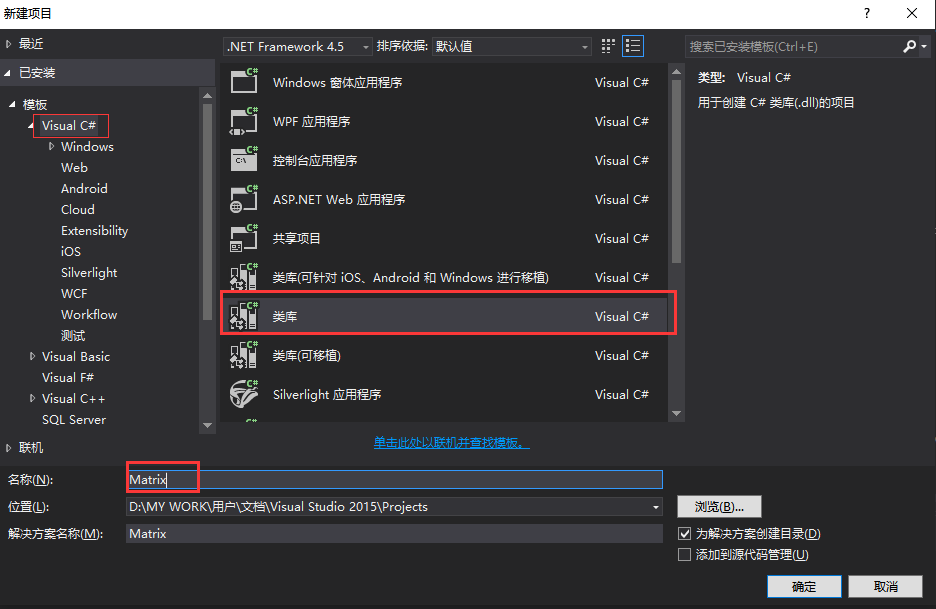2 然后把代码（代码需下载，见于博客最后链接）粘到里面，点击生成->生成解决方案。
注意：这个粘指的是完全粘，骨头渣都不剩的那种，具体操作可以参考：

1.打开下载的代码
Ctrl+A,Ctrl+C

2.回到新建的 Matrix 项目
Ctrl+A,Ctrl+V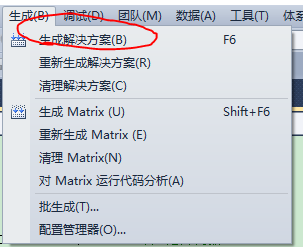之后在debug 目录下看到 .dll 文件：（补于2020/6/26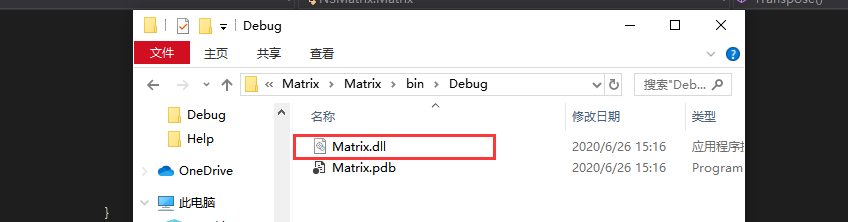2.3 将debug 目录下的“Matrix.dll” 复制到所需矩阵运算的项目 debug 目录
然后在项目 引用中添加引用：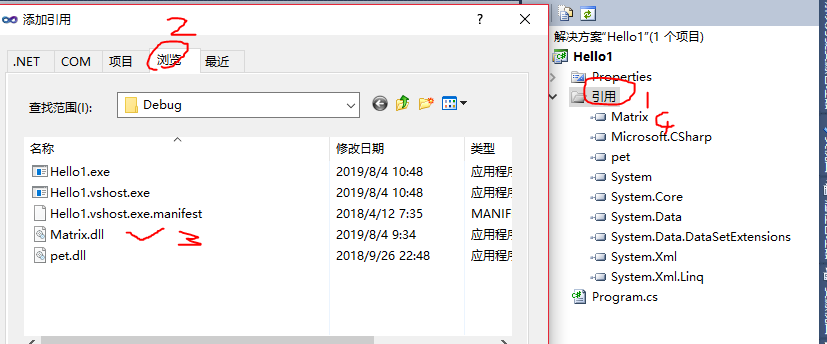2.4 在文件头添加：
using NSMatrix;
2020/6/19 ：添加使用方法2

### 方法2：不需.dll

有的朋友反映生成不了.dll （今天我用VS2015做了个实验，发现确实（实际上是操作不当😂😂），在这里给各位道个歉，之前没有做足实验，给您带来不便，着实抱歉！！）所以今天补一个不需要.dll 的使用方法。（ps:我猜好像vs版本高于2010就不可以生成.dll了，也有可能我们生成.dll的方法不对）

1.新建个你的项目（也就是你要进行矩阵运算的那个项目，也许你的项目已经存在，这里只是为了更好说明情况，新建了个项目，名字叫做：Hello10086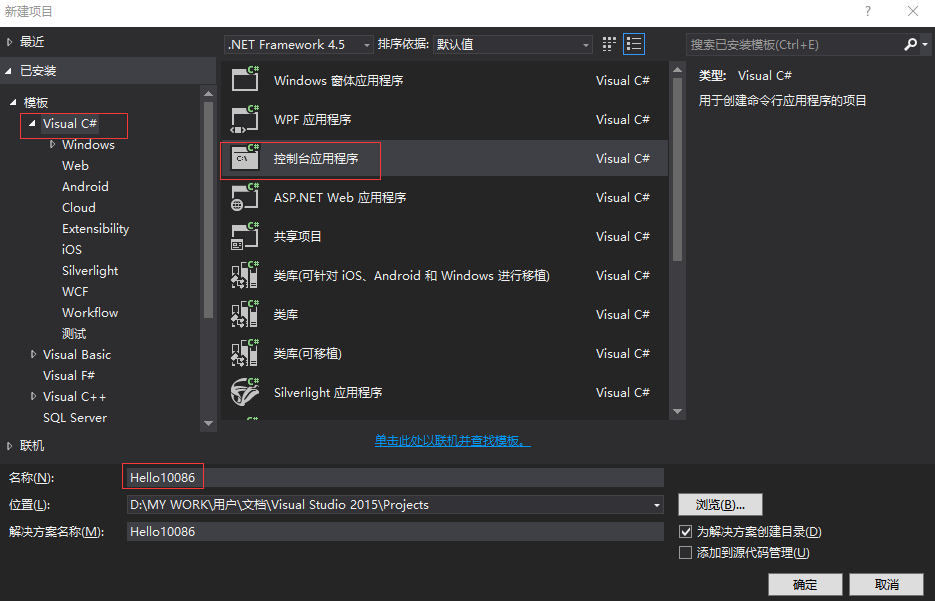之后生成的文件里面内容如下：

using System;
using System.Collections.Generic;
using System.Linq;
using System.Text;

namespace Hello10086//这个namespace 是你的项目的namespace
{
class Program
{
static void Main(string[] args)
{
}
}
}


2.新建个类文件，名字随便取，这里取成了Matrix.cs，之前有自己项目的，直接来这一步。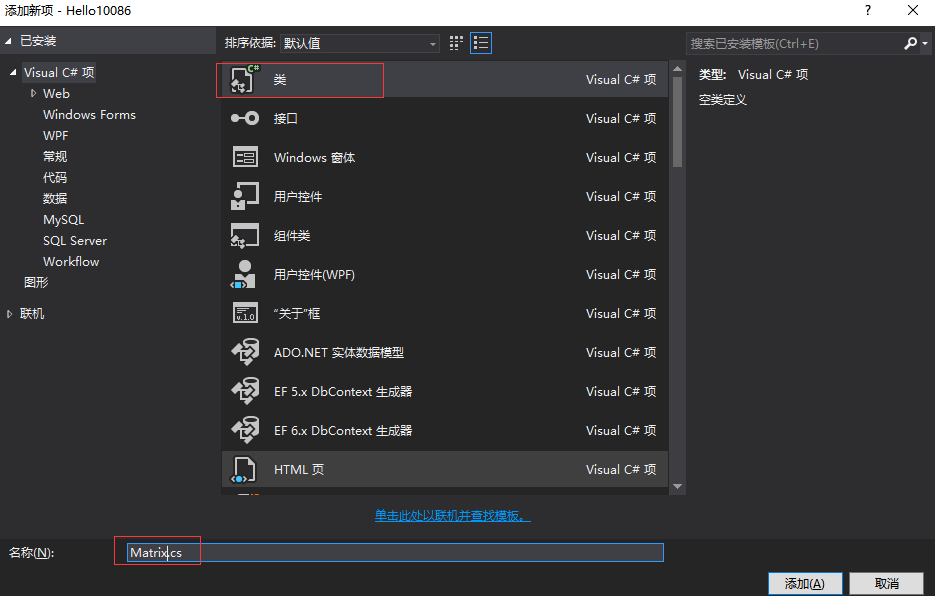3.把下载的代码贴到里面，然后将命名空间（namespace）改成你的项目的命名空间：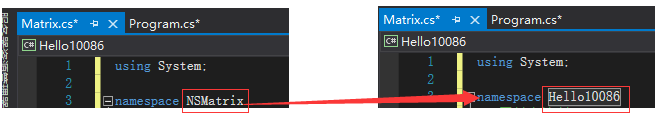4.之后可以用下面的使用示例跑一下看看。我今天这个实验没问题。

注意：贴使用示例的时候，只需把Main 中的代码提到你的Main中，其他的不需贴。

厚着脸皮求个赞：看到这里的朋友可不可以来个关注或小赞，您的欣赏是对我最大的支持和鼓励！

## 3. 使用示例：

using System;
using System.Collections.Generic;
using System.IO;
using System.Text;
using System.Linq;
using System.Data.OleDb;

using NSMatrix;

namespace Test2017
{
class Program
{
static void Main(string[] args)
{
double[] A_data ={ 1, 2, 8, 4, 5, 6, 7, 8, 9 };
double[,] B_data = { {1, 2, 3},{ 4, 5, 6}, {7, 8, 9 }};
Matrix A=new Matrix(3,3,A_data);
Matrix B = new Matrix(B_data);
Console.WriteLine("A:");
A.Show_Mat();
Console.WriteLine("B:");
B.Show_Mat();
Console.WriteLine("A+B:");
(A + B).Show_Mat();
Console.WriteLine("A-B:");
(A - B).Show_Mat();
Console.WriteLine("A*B:");
(A * B).Show_Mat();
Console.WriteLine("A的转置:");
(A.Transpose()).Show_Mat();
Console.WriteLine("A的行列式:");
Matrix C = new Matrix(A);//由于使用下面的函数后，矩阵会变
Console.WriteLine("{0}",C.ComputeDetGauss());
Console.WriteLine("A的逆:");
A.InvertGaussJordan();
A.Show_Mat();
Console.WriteLine("变值后的B:");
B[0, 1] = 8;
B.Show_Mat();
}
}
}


结果：

A:
1.000          2.000          8.000
4.000          5.000          6.000
7.000          8.000          9.000
B:
1.000          2.000          3.000
4.000          5.000          6.000
7.000          8.000          9.000
A+B:
2.000          4.000         11.000
8.000         10.000         12.000
14.000         16.000         18.000
A-B:
0.000          0.000          5.000
0.000          0.000          0.000
0.000          0.000          0.000
A*B:
65.000         76.000         87.000
66.000         81.000         96.000
102.000        126.000        150.000
A的转置:
1.000          4.000          7.000
2.000          5.000          8.000
8.000          6.000          9.000
A的行列式:
-15
A的逆:
0.200         -3.067          1.867
-0.400          3.133         -1.733
0.200         -0.400          0.200
变值后的B:
1.000          8.000          3.000
4.000          5.000          6.000
7.000          8.000          9.000



感兴趣的朋友可以下载~
特别感谢编制这个矩阵库的前辈，本人学到了很多！->_<-展开全文• 一、矩阵求逆算法 二、矩阵求逆的代码实现

# 一、矩阵求逆算法

## 本次实现矩阵求逆的算法为伴随矩阵法

代数余子式求逆矩阵：如果矩阵A可逆，则
A − 1 = A ∗ ∣ A ∣ A^{-1} = \frac{A^{*}}{|A|}
(|A| != 0) ,|A|为该矩阵对应的行列式的值

设三阶矩阵A
A = [ a b c d e f g h i ] A=\left[ \begin{matrix} a & b & c \\ d & e & f \\ g & h & i \end{matrix} \right]

1、首先求出伴随矩阵
A ∗ = [ e i − h f h c − b i b f − c e f g − i d i a − c g c d − a f d h − g e g b − a h a e − b d ] A^{*}=\left[ \begin{matrix} ei-hf & hc-bi & bf-ce \\ fg-id & ia-cg & cd-af \\ dh-ge & gb-ah & ae-bd \end{matrix} \right]
将矩阵 A 中的数值带入即可得出伴随矩阵

2、求出其行列式的值
∣ A ∣ = a e i + b f g + c d h − c e g − b d i − a f h |A| = aei + bfg+cdh-ceg-bdi-afh
3、将结果带入式
A − 1 = A ∗ ∣ A ∣ A^{-1} = \frac{A^{*}}{|A|}
中即可得出矩阵的逆

# 二、矩阵求逆的代码实现

1、按照上述方式，首先求出矩阵的伴随矩阵

首先定义一个3X3的二维数组表示一个矩阵

 double[,] input = new double[3, 3];   //初始矩阵
double[,] output = new double[3, 3];  //矩阵的逆


———求出伴随矩阵——

 output[0, 0] = input[2, 2] * input[1, 1] - input[2, 1] * input[1, 2];
output[0, 1] = input[2, 1] * input[0, 2] - input[0, 1] * input[2, 2];
output[0, 2] = input[0, 1] * input[1, 2] - input[0, 2] * input[1, 1];

output[1, 0] = input[1, 2] * input[2, 0] - input[2, 2] * input[1, 0];
output[1, 1] = input[2, 2] * input[0, 0] - input[0, 2] * input[2, 0];
output[1, 2] = input[0, 2] * input[1, 0] - input[0, 0] * input[1, 2];

output[2, 0] = input[1, 0] * input[2, 1] - input[2, 0] * input[1, 1];
output[2, 1] = input[2, 0] * input[0, 1] - input[0, 0] * input[2, 1];
output[2, 2] = input[0, 0] * input[1, 1] - input[1, 0] * input[0, 1];


———————求出行列式的值———————

 double Avalue   = input[0, 0] * input[1, 1] * input[2, 2]
+ input[0, 1] * input[1, 2] * input[2, 0]
+ input[0, 2] * input[1, 0] * input[2, 1]
- input[0, 2] * input[1, 1] * input[2, 0]
- input[0, 1] * input[1, 0] * input[2, 2]
- input[0, 0] * input[1, 2] * input[2, 1];



———————求出 逆矩阵 ———————

            for (int i = 0; i < 3; i++)
{
for (int j = 0; j < 3; j++)
{
output[i, j] = output[i, j] / Avalue;
}
}


得出 output[ i , j ] 即为**矩阵的逆**

## 以下完整代码

public static double[,] inv3(double[,] input)
{

double[,] output = new double[3, 3];

output[0, 0] = input[2, 2] * input[1, 1] - input[2, 1] * input[1, 2];
output[0, 1] = input[2, 1] * input[0, 2] - input[0, 1] * input[2, 2];
output[0, 2] = input[0, 1] * input[1, 2] - input[0, 2] * input[1, 1];

output[1, 0] = input[1, 2] * input[2, 0] - input[2, 2] * input[1, 0];
output[1, 1] = input[2, 2] * input[0, 0] - input[0, 2] * input[2, 0];
output[1, 2] = input[0, 2] * input[1, 0] - input[0, 0] * input[1, 2];

output[2, 0] = input[1, 0] * input[2, 1] - input[2, 0] * input[1, 1];
output[2, 1] = input[2, 0] * input[0, 1] - input[0, 0] * input[2, 1];
output[2, 2] = input[0, 0] * input[1, 1] - input[1, 0] * input[0, 1];

double Avalue = input[0, 0] * input[1, 1] * input[2, 2]
+ input[0, 1] * input[1, 2] * input[2, 0]
+ input[0, 2] * input[1, 0] * input[2, 1]
- input[0, 2] * input[1, 1] * input[2, 0]
- input[0, 1] * input[1, 0] * input[2, 2]
- input[0, 0] * input[1, 2] * input[2, 1];

for (int i = 0; i < 3; i++)
{
for (int j = 0; j < 3; j++)
{
output[i, j] = output[i, j] / Avalue;
}
}
return output;

}

展开全文• c#初级学习者有很大的帮助，在应用到之后的算数编程有很好的辅助功能。
• 输入行和列，再输入对应的数字，然后求和，思路很清晰

牛客网上的一道题，题目要求我就重复说了，大家看一下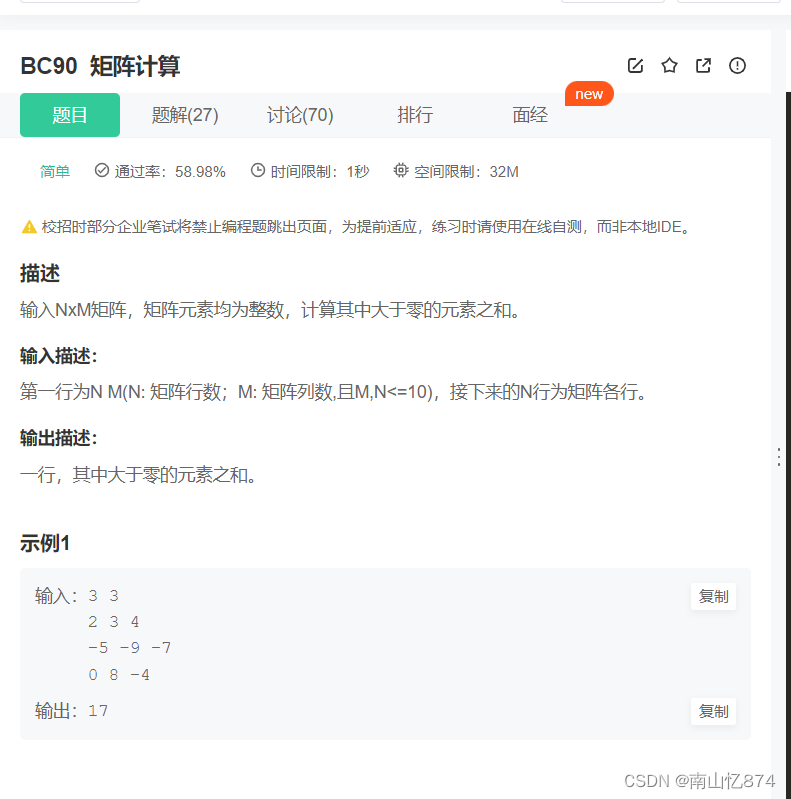其实这道题目不难，两个循环就搞定了，直接给大家上代码吧

#include <stdio.h>

int main()
{
int arr = { 0 };

int n, m;
scanf("%d %d", &n, &m);

int i = 0;
int j = 0;
for (i = 0; i < n; i++)
{
for (j = 0; j < m; j++)
{
scanf("%d", &arr[i][j]);
}
}
int sum = 0;
for (i = 0; i < n; i++)
{
for (j = 0; j < m; j++)
{
if (arr[i][j] > 0)
{
sum += arr[i][j];
}
}
}
printf("%d", sum);

return 0;
}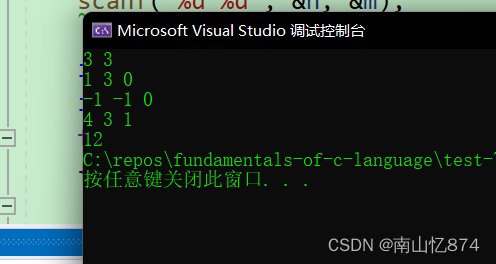有不懂的可以给我留言，关于C的其他问题也可以留言哦

展开全文• 主要介绍了C#计算矩阵的秩实现方法,以实例形式较为详细的分析了C#计算矩阵秩的原理与实现技巧,具有一定参考借鉴价值,需要的朋友可以参考下
• } // 矩阵的创建 Matrix A = Matrix.Create(4, 5, d1); Matrix B = Matrix.Create(4, 5, d2); Matrix Mat = Matrix.Create(2, 2, new double[] { 1, 2, 3, 4 }); // 矩阵的深度复制 Matrix C = new Matrix(A....
• } else { //在矩阵右方增加一同型单位矩阵 for (int i = 0; i ; i++) { for (int j = 0; j ; j++) { if (j ) subMatrix[i, j] = Element[i, j]; else { if (i == j - Columns) subMatrix[i, j] = 1; else subMatrix...
• C#矩阵运算demo,带测试例子，可以直接使用。c# 矩阵
• 在学习吴恩达教授机器学习时，其中讲解到关于矩阵向量部分。由此记录。 概念 矩阵实例 下面的矩阵用A来代替 向量 向量和矩阵相乘时，用矩阵的每一行去和向量的每一列相乘 即得到的第一个值为 a1 * b1+a2 * b2. ...机器学习
• C# 矩阵算法 .net 矩阵算法。C#矩阵运算类。实现转置,相乘,相加,求逆。
• 本文实例讲述了C#实现矩阵加法、取负、数乘、乘法的方法。分享给大家供大家参考。具体如下： 1.几个基本函数 1）判断一个二维数组是否为矩阵：如果每行的列数都相等则是矩阵，没有元素的二维数组是矩阵 /// /// ...
• 功能：可以进行矩阵转置，矩阵相乘，矩阵相加，矩阵求逆。 输入输出： 用户可以输入矩阵值后，可以选择矩阵运算类型，每次运算后都要输出运算后的矩阵。 若有错误，提示错误。
• 主要介绍了C#实现矩阵转置的方法,实例分析了C#针对矩阵运算的相关技巧,具有一定参考借鉴价值,需要的朋友可以参考下C# 矩阵转置
• ## C#矩阵运算类

千次阅读 2019-12-25 22:26:39
命名空间：matrix 定义类：Matrix ...定义矩阵A: Matrix A=new Matrix(int mm, int nn); public double read(int i, int j) 获取Aij: A.read(i,j); public int write(int i, int j, double val) 将数据...
• ## C#矩阵乘法

千次阅读 2018-11-27 22:12:02
///<summary> ///<summary> ///矩阵乘法 ///</summary> ///<paramname=”matrix1″>矩阵1</param> ///<paramname=”ma...
• C# 编写矩阵 重载运算符 实现矩阵的相加、减，相乘C# 矩阵
• ## C#矩阵相加代码

千次阅读 2019-11-19 21:29:33
两个矩阵相加的过程，并显示两个矩阵相加后的结果： using System; namespace MatrixAdd { class Program { static void MatrixAdd(int[,] arrA, int[,] arrB, int[,] arrC, int dimX, int dimY) { int...
• ## C#矩阵相乘

千次阅读 2018-09-20 15:46:49
/// 矩阵乘法 /// &lt;param name="matrix1"&gt;矩阵1&lt;/param&gt; /// &lt;param name="matrix2"&gt;矩阵2&lt;/param&gt; /// &lt;...
•C# 矩阵乘法
• C#本身不支持矩阵，所以不适合数值运算，但是C#的优点又在于界面编写简单，如果需要用到界面，且必须用到矩阵，那么就就需要引用一个矩阵类...本次矩阵类主要是基于Python以及Matlab矩阵的格式规范，轻松上手C#矩阵编写C#矩阵...